﻿ 一种带约束限制的三次B样条曲线矢量数据压缩算法
数学杂志2021, Vol. 41Issue (3): 247-256PDF
 扩展功能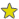加入收藏夹复制引文信息加入引用管理器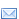Email AlertRSS 本文作者相关文章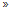冯峰蒋维

A CUBIC B-SPLINE-BASED VECTOR DATA COMPRESSION ALGORITHM WITH BOUNDARY CONSTRAINTS
FENG Feng, JIANG Wei
School of Mathematics and Statistics, Wuhan University, Wuhan 430072, China
Abstract: In order to efficiently retrieve, analyze, store and transmit large amount of vector data, it is extremely necessary to compress these vector data in advance. Based on elegant properties of the B-spline (e.g., locality and smoothness), we propose a cubic B-spline-based algorithm to compress the vector data with boundary constraints. The proposed cubic B-spline vector data compression algorithm is tested on nine examples with curve vector data. We also compare numerical results produced by the proposed algorithm with these of the classical Douglas-Peucker compression algorithm. Numerical results show that the proposed cubic B-spline-based vector compression algorithm not only can significantly reduce the compression rate, but also can produce highly accurate compression curve with C2-smoothness. Therefore, the algorithm has many important potential applications (e.g., automatic drive).
Keywords: curve vector data compression     cubic B-spline     C2-smoothness     Douglas-Peucker algorithm     boundary constraints
1 引言

 $\begin{equation} \mathcal{P} = \left\{ {\bf{P}}_{1}, {\bf{P}}_{2}, {\bf{P}}_{3}, \ldots, {\bf{P}}_{|\mathcal{P}|-1}, {\bf{P}}_{|\mathcal{P}|} \right\}, \end{equation}$ (1.1)

 $\begin{equation} \mathcal{P}^{\prime} = \left\{ {\bf{P}}_{1}^{\prime}, {\bf{P}}_{2}^{\prime}, {\bf{P}}_{3}^{\prime}, \ldots, {\bf{P}}_{|\mathcal{P^\prime}|-1}^{\prime}, {\bf{P}}_{|\mathcal{P^\prime}|}^{\prime} \right\}, \end{equation}$ (1.2)

 $\begin{equation} \alpha = \frac{|\mathcal{P^\prime}|}{|\mathcal{P}|}, \end{equation}$ (1.3)

2 B样条曲线简介

B样条曲线是一组分段多项式基函数的线性组合, 而这些基函数确定了曲线的连续性. 具体来说, 一条平面上的B样条曲线$\bf{X}: \mathbb{R} \rightarrow \mathbb{R}^2$, i.e., $\rho \mapsto \bf{X}(\rho)$可以表示为

 $\begin{equation} {\bf{X}}(\rho) = \sum\limits_{i = 0}^n {\bf{P}}_i N_{i, k}(\rho), \end{equation}$ (2.1)

 $\begin{equation} {N_{i, k}(\rho) = \frac{\rho-\rho_{i}}{\rho_{i+k}-\rho_{i}} N_{i, k-1}(\rho)+\frac{\rho_{i+k+1}-\rho}{\rho_{i+k+1}-\rho_{i+1}} N_{i+1, k-1}(\rho)}, \end{equation}$ (2.2)

 $\begin{equation} {\bf{X}}^{(r)}(\rho) = \sum\limits_{i = 0}^n {\bf{P}}_i N_{i, k}^{(r)}(\rho). \end{equation}$ (2.4)

3 三次B样条曲线拟合

 $\begin{equation} \min \ \sum\limits_{k = 0}^{K}\left|{\bf{Q}}_{k}-{\bf{X}}\left(\overline{\rho}_{k}\right)\right|^{2}, \end{equation}$ (3.1)

$\overline{\rho}_{k}$为预先给定的数据点参数值, 这里我们利用积累弦长法确定, 即有

 $\begin{equation} \overline{\rho}_k = \overline{\rho}_{k-1}+\frac{\left|{\bf{Q}}_k-{\bf{Q}}_{k-1}\right|}{\sum\limits_{i = 1}^{K}\left|{\bf{Q}}_i-{\bf{Q}}_{i-1}\right|} , \ k = 1, \ldots, K-1, \end{equation}$ (3.2)

 $\begin{equation} {\bf{X}}(0) = {\bf{P}}_{0}, \qquad {\bf{X}}(1) = {\bf{P}}_{n}. \end{equation}$ (3.3)

 $\begin{equation} \min\limits_{\bf{P}} \ {\textbf{tr}}\bigl[({\bf{Q}}-{\bf{N}}{\bf{P}})^T({\bf{Q}}-{\bf{N}}{\bf{P}})\bigr], \end{equation}$ (3.4)

 $\begin{equation} \min\limits_{\bf{P}}\, \textbf{tr}\bigl[ \left({\bf{Q}}-{\bf{N}}{\bf{P}}\right) ^T \left({\bf{Q}}-{\bf{N}}{\bf{P}}\right)+{\bf{A}}^{T}({\bf{M}} {\bf{P}}-{\bf{T}})\bigr]. \end{equation}$ (4.6)

 $\begin{equation} {\bf{P}} = \left({\bf{N}}^{T} {\bf{N}}\right)^{-1} {\bf{N}}^{T} {\bf{Q}}-\left({\bf{N}}^{T} {\bf{N}}\right)^{-1} {\bf{M}}^{T} {\bf{A}}, \end{equation}$ (4.9)
 $\begin{equation} {\bf{A}} = ({\bf{M}}\left({\bf{N}}^{T}{\bf{N}}\right)^{-1} {\bf{M}}^{T})^{-1}({\bf{M}}\left({\bf{N}}^{T} {\bf{N}}\right)^{-1} {\bf{N}}^{T} {\bf{Q}}-{\bf{T}}). \end{equation}$ (4.10)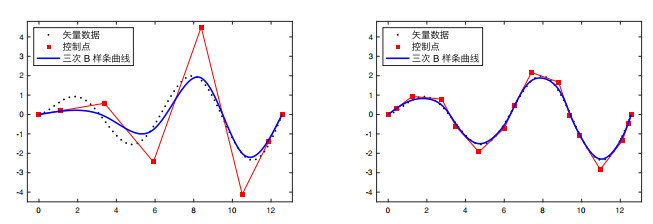图 4 带约束条件限制的三次B样条曲线拟合, 左图: 8个控制点; 右图: 16个控制点.

(1) 利用积累弦长法(3.2) 将曲线矢量数据参数化$\{\left({\bf{Q}}_k, \ \overline{\rho}_{k}\right)\}_{k = 0}^K$.

(2) 给定误差阈值$\varepsilon$, 选取三次B样条初始控制点数目$n$ (这里的$n$值初始选取不应过大)求解带等式约束的二次规划问题, 由式(4.9) 得到相应的控制点序列, 其中三次B样条的节点向量设定为准均匀分布.

(3) 计算所有数据点到拟合的三次样条曲线的误差, 如果数据点到样条曲线的最大误差小于给定误差阈值$\varepsilon$:

 $\begin{equation} \max \limits_{k = 0, \ldots , K}\left|{\bf{Q}}_{k}-{\bf{X}}\left(\overline{\rho}_{k}\right)\right| < \varepsilon, \end{equation}$ (4.11)5 数值结果比较

 $\begin{equation} \alpha_{\rm{bsp}} = \frac{\rm{三次\; B\; 样条曲线中控制点数目}}{\rm{曲线矢量数据中矢量点数目}}, \end{equation}$ (5.1)

 $\begin{equation} \alpha_{\rm{dp}} = \frac{\rm{压缩后剩余矢量点数目}}{\rm{曲线矢量数据中矢量点数目}}. \end{equation}$ (5.2)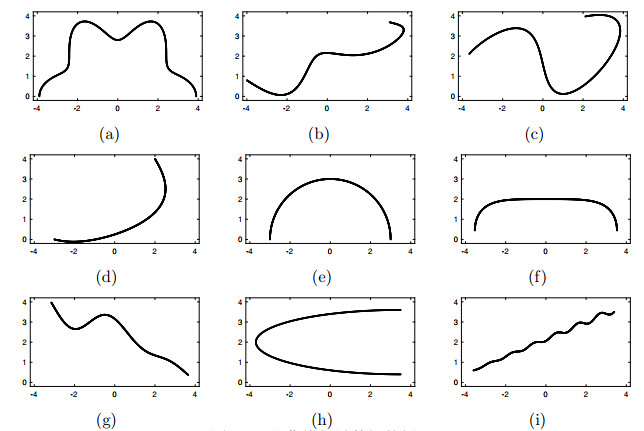图 5 一些曲线矢量数据的例子表 1 Douglas-Peucker算法的压缩率αdp数值结果表 2 基于三次样条压缩算法的压缩率αbsp的数值结果
6 结论

  温永宁, 等. 矢量空间数据渐进传输研究进展[J]. 地理与地理信息科学, 2011, 27(6): 6–12.  张振鑫, 张维, 刘嫔, 等. 矢量地图数据简化研究进展[J]. 测绘工程, 2016, 25(6): 10–14. DOI:10.3969/j.issn.1006-7949.2016.06.003  杨建宇, 杨崇俊, 明冬萍, 等. WebGIS系统中矢量数据的压缩与化简方法综述[J]. 计算机工程与应用, 2005, 40(32): 36–38.  Douglas D, Peucker T. Algorithms for the reduction of the number of points required to represent a line or its caricature[J]. The Canadian Cartographer, 1973, 10(2): 112–122. DOI:10.3138/FM57-6770-U75U-7727  Li Z, Openshaw S. Algorithms for automated line generalization based on a natural principle of objective generalization[J]. International Journal of Geographical Information Systems, 1992, 6(5): 373–389. DOI:10.1080/02693799208901921  黄培之. 具有预测功能的曲线矢量数据压缩方法[J]. 测绘学报, 1995, 024(4): 316–320.  马伯宁, 冷志光, 汤晓安, 等. 具有误差修正的线矢量数据小波变换[J]. 计算机辅助设计与图形学学报, 2011(11): 1825–1829.  吴凡. 基于小波分析的线状特征数据无级表达[J]. 武汉大学学报(信息科学版), 2004, 29(6): 488–491.  单玉香. 矢量数据压缩模型与算法的研究[D]. 太原理工大学, 2004.  Piegl L, Tiller W. The NURBS book[M]. Berlin: Springer, 1997.  De Boor C. A practical guide to splines, volume 27[M]. New York: Springer-verlag, 1978.  Kincaid D, Cheney W. Numerical analysis: mathematics of scientific computing, volume 2[M]. American Mathematical Society, 2009.  施法中. 计算机辅助几何设计与非均匀有理B样条[M]. 高等教育出版社, 2001.  Unser M, Aldroubi A, Eden M. B-spline signal processing. i. theory[J]. IEEE Transactions on Signal Processing, 1993, 41(2): 821–833. DOI:10.1109/78.193220  Unser M, Aldroubi A, Eden M. B-spline signal processing. ii. efficiency design and applications[J]. IEEE Transactions on Signal Processing, 1993, 41(2): 834–848. DOI:10.1109/78.193221  Unser M. Splines: A perfect fit for signal and image processing[J]. IEEE Signal Processing Magazine, 1999, 16(6): 22–38. DOI:10.1109/79.799930